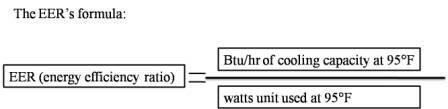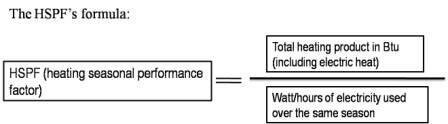## What is SEER, EER, HSPF, AFUE, and COP?

What does SEER, EER, HSPF, AFUE and COP stand for?

What does the energy efficiency rating number mean in central air conditioner, window air conditioner, heat pump, or furnace units?

These energy efficiencies stand for:

• Seasonal Energy Efficiency Ratio
• Energy Efficiency Rating Ratio
• Heating Seasonal Performance Factor
• Annual Fuel Utilization Efficiency Rating
• Coefficient of Performance

Why does a HVAC (Heating, Ventilating, and Air Conditioning) unit have energy efficiency measurement?

The Air Conditioning and Refrigeration Institute (ARI) adapted energy efficiency rating for sole purpose of saving energy!

They needed a universal standard of measurement to determine the energy efficiency of HVAC units.

These measurements are in SEER (central air conditioner units, Heat Pump cooling season), EER (window unit), HSPF (Heat Pump), AFUE (Furnace, boilers) and COP (heat pump, chiller).

The higher the measurement of energy efficiency rating is, the more energy you save!

What is Seasonal Energy Efficiency Ratio?

It is the efficiency air conditioner manufacture rate a central air conditioner with. It is measurement of central air conditioners or heat pump usage over the cooling season.

It is the total supply cooling of an air conditioner Btu you get out; during its normal usage periods and compared it to electricity energy the central air conditioners consumed (watt/hr) in that same cooling season and you get SEER.

The seasonal energy efficiency ratio is express in Btu/watt-hour

11 Seer = 11 Btu/watt-hour

For instance, central air conditioner units that have rate of 11 SEER will produce 11Btu of cooling per watt-hour (WH).OR

BTU ÷ W•h = SEER

Let calculate few math by using information below.

For example, let’s say you have a central air conditioner that is 36000 BTU/h (3 tons), it is 11 SEER units, and you plan to run for 8 hours each day for 4 months in the summer ( let’s presume that a summer about 4 months, and there are 30 days in month). The electricity cost in kilowatt-hour in your area about \$0.06.

For 4 months, central air conditioning unit will run 960 hours.

4 months x 30 days/month x 8 hr/day = 960 hours

That central air conditioner unit will produce about 34,560,000 BTU for 4 months.

36,000 Btu/h x 960h = 34,560,000 Btu

11 SEER central air conditioner units will use about 3,141,818 Watt-hour for 4 months.

34,560,000 Btu / 11 Btu/watt-hour = 3, 141, 818 watt-hour

How much electricity energy does a 36,000 Btu/h central air conditioner units use per-hour?

Here how to calculate electrical energy using per hour. You divide 36, 000 Btu/h with 11 Seer (Btu/watt-hours). (36,000 Btu/h) / (11 Btu/watt-hours) = about 3272.73 watt.

It costs about \$188.51 to run a central air conditioner with electrical cost in kilowatt-hour \$.06 for 4 months.

36,000 BTU/hr x 960 hours / 11 SEER = 3141818.2

\$0.06 kilowatt-hour/1000 watts/kilowatt = 6x10^ (-5)

(3141818.2) X (6x10^ (-5)) = \$188.51 to run central air conditioner units for 4 months.

Here is Seasonal Energy Efficiency Ratio and HSPF Video

HVAC Training Videos made by alpinehomeair

Make sure to press the Play button in the player controls to watch it. Enjoy!

What is an energy efficiency ratio or EER?

Energy Efficiency Ratio (EER) is a measurement of the cooling capacity for a unit (in Btu/hour) divided by electrical energy it uses (in watts) at a specific temperature of 95°F.

The energy efficiency ratios are normally used with a window unit or geothermal heat pumps.For example, let said you have a window air conditioner unit that has a cooling capacity of 11,000 Btu per hour and the outdoor temperature is 95°F. The window unit is consuming 1000 watts of electricity; it would have an energy efficiency rating of 11.

The higher the energy efficiency rating is, the less energy the window air conditioner units use and the more money you save on your utility bill.

What is a Heating Seasonal Performance Factor or HSPF?

Heat Pumps are rate with two energy efficiency: HSPF is a measurement of Heat pump’s energy efficiency over one heating season and the cooling efficiency of Heat Pump is measuring with Seasonal Energy Efficiency Rating over one cooling season.

Heating Seasonal Performance Factor is total heating output (supply heat) in Btu (including electric heat) during heating season and divided to the total electricity energy heat pump consumed (in watt/hr) in the same season and you get HSPF.What is Coefficient of Performance or COP?

Coefficient of performance is the ratio of output energy divided by the input energy. It is normally used to measure how efficiency heating and cooling in heat pump or chiller for a cooling mode.

The example below is for cooling capacity.What is Annual Fuel Utilization Efficiency Rating or AFUE?

Annual Fuel Utilization Efficiency is a measurement to determine the efficiency of a furnace and boilers. It is a seasonal energy efficiency of fuel heating equipment.

AFUE pronounced as (ah) ‘Few’ or (A) ‘Few’

Annual fuel utilitzation efficiency is a rating that shows how efficient a gas furnace converts fuel to space heat (through combustion) in comparison to the amount of fuel the furnace used. It expressed as a percentage.

Annual fuel utilitzation efficiency doe not refers to electrical usage (blower, fan or any electrical parts) in a furnace. It is fuel efficiency measurement for furnace or boilers.

These standards of measurement tell us the efficiency of HVAC units. Every HVAC units have it. This efficiency number is what you compare to buy the best air conditioner for your home.

Return from SEER, EER, HSPF, AFUE and COP to Central Air Conditioner and Refrigeration